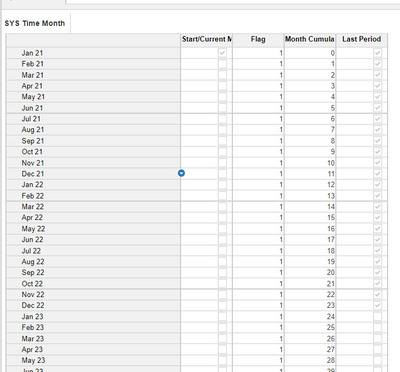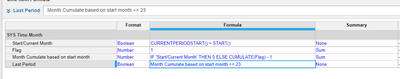# Formula to end timeHi I have this formula that will add 1(digit) to the months following the current start month however I would like it to stop adding number after month 23. I can't figure out how to end the argument/formula so it stops adding number.

Ex: Current month is 0, Next month is 1, following month is 2 etc, last month should be 23. How do I get it to stop counting after 23

IF ITEM(Time) = 'SYS02 Filter - Input'.'Start Month (F. Export)' THEN 0 ELSE IF ITEM(Time) > 'SYS02 Filter - Input'.'Start Month (Synergi Export)' THEN PREVIOUS(Step) + 1 ELSE 0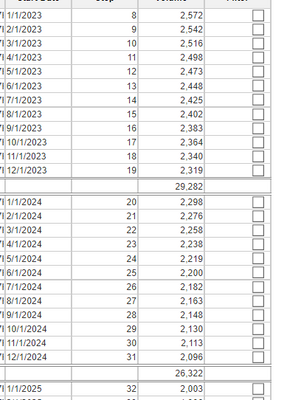Tagged:

•Hi @umandiwa ,

There is a different way to calculate to stop the period, as of now i can think of below approach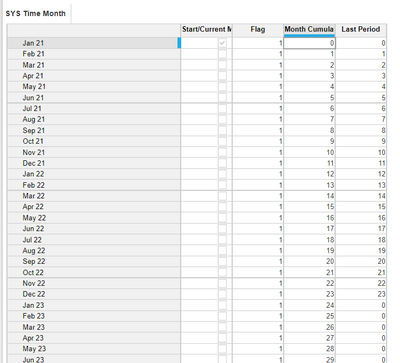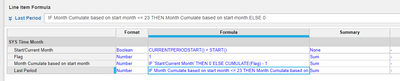You can use last period as your filter also.

Hope this will help

Thanks

Akhtar

•If I were new to Anaplan, I would have created something like this:

IF ITEM(Time) = 'SYS02 Filter - Input'.'Start Month (F. Export)' THEN 0 ELSE IF ITEM(Time) > 'SYS02 Filter - Input'.'Start Month (Synergi Export)' THEN IF PREVIOUS(Step) <= 22 THEN PREVIOUS(Step) + 1 ELSE 0 ELSE 0

To make it slightly better, I would have a separate system module without any dimension that will take the number 22 as a user input in consonance with PLANS and then refer that instead of hard coding the number 22.

Not sure if you can, but try to limit the number of IF/THEN/ELSE loops that you have

Let me know if that helps

•